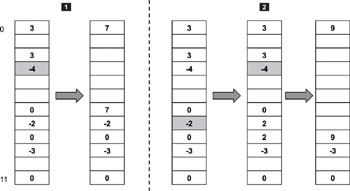home | login | register | DMCA | contacts | help | donate |A B C D E F G H I J K L M N O P Q R S T U V W X Y Z
А Б В Г Д Е Ж З И Й К Л М Н О П Р С Т У Ф Х Ц Ч Ш Щ Э Ю Я

# 13.2.4 The free Operation

Note that the bottom layer of the malloc and free implementation is shown in Figure 13.3 and Figure 13.4. In other words, another layer of software tracks, for example, the address of an allocated block and its size. Let's assume that this software layer exists and that the example is not concerned with it other than that this layer feeds the necessary information into the free function.

The main operation of the free function is to determine if the block being freed can be merged with its neighbors. The merging rules are

1. If the starting index of the block is not 0, check for the value of the array at (index -1). If the value is positive (not a negative value or 0), this neighbor can be merged.

2. If (index + number of blocks) does not exceed the maximum array index value, check for the value of the array at (index + number of blocks). If the value is positive, this neighbor can be merged.

These rules are illustrated best through an example, as shown in Figure 13.5.Figure 13.5: The free operation.

Figure 13.5 shows two scenarios worth discussion. In the first scenario, the block starting at index 3 is being freed. Following rule #1, look at the value at index 2. The value is 3; therefore, the neighboring block can be merged. The value of 3 indicates that the neighboring block is 3 units large. The block being freed is 4 units large, so following rule #2, look at the value at index 7. The value is -2; therefore, the neighboring block is still in use and cannot be merged. The result of the free operation in the first scenario is shown as the second table in Figure 13.5.

In the second scenario, the block at index 7 is being freed. Following rule #1, look at the value at index 6, which is 0. This value indicates the neighboring block is still in use. Following rule #2, look at the value at index 9, which is -3. Again, this value indicates that this block is also in use. The newly freed block remains as independent piece. After applying the two merge rules, the next free operation of the block starting at index 3 results in the allocation table shown as the last table in Figure 13.5.

When a block is freed, the heap must be updated accordingly. Therefore, the free operation involves the following steps:

1. Update the allocation array and merge neighboring blocks if possible.

2. If the newly freed block cannot be merged with any of its neighbors, insert a new entry into the heap array.

3. If the newly freed block can be merged with one of its neighbors, the heap entry representing the neighboring block must be updated, and the updated entry rearranged according to its new size.

4. If the newly freed block can be merged with both of its neighbors, the heap entry representing one of the neighboring blocks must be deleted from the heap, and the heap entry representing the other neighboring block must be updated and rearranged according to its new size.

13.2.3 Finding Free Blocks Quickly | Real-Time Concepts for Embedded Systems | 13.3 Fixed-Size Memory Management in Embedded Systems